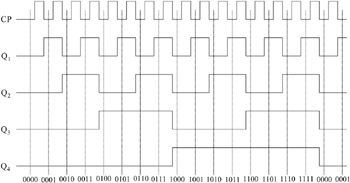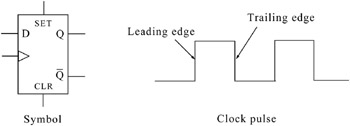Digital Circuit Design with an Introduction to CPLDs and FPGAs

# 8.3: Data (D) Flip Flop

## 8.3 Data (D) Flip Flop

The data (D) flip flop is a modification of a synchronous (clocked) SR flip flop the latter of which can be constructed either with NAND or with NOR gates. Figure 8.5 shows the construction of a typical clocked D flip flop with NAND gates, and Table 8.3 its characteristic table.Figure 8.5: Construction of a D flip flop
Table 8.3: Characteristic table for the D flip flop with NAND gates

Input

Present State

Next State

D

Q n

Q n+1

0

0

0

0

1

0

1

0

1

1

1

1

The characteristic table in Table 8.3 shows that the next state Q n+1 is the same as the input D, and for this reason the D flip flop is often called a data transfer flip flop. Its symbol is shown in Figure 8.26 where the small triangle inside the rectangle is used to indicate that this type of flip flop is triggered during the leading edge of the clock pulse and this is normally indicated with the pulse shown in Figure 8.6.Figure 8.26: Timing diagram for the circuit of Figure 8.25Figure 8.6: D flip flop symbol activated during the leading edge of the clock pulse

Device SN7474 is a D flip flop with asynchronous Set and Clear commands.